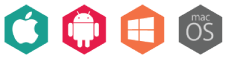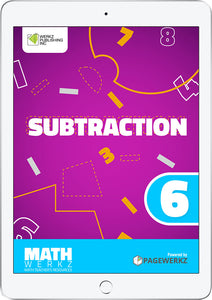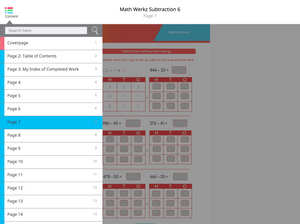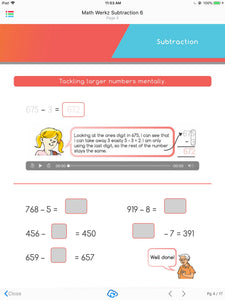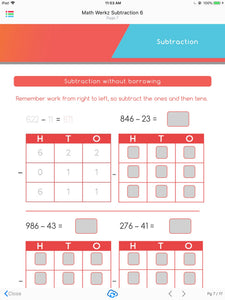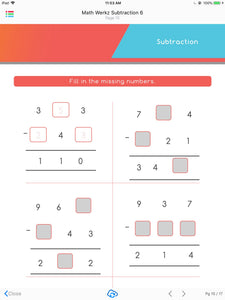# Math Werkz Subtraction 6

Regular price \$2.00 Sale

Product Description:

Math Werkz Subtraction 6 is a fun, interactive courseware that teaches kids about subtraction.

This interactive eBook includes worksheets and fun activities which allow children to know more about subtracting numbers through the use of multiples, number lines, and word problems. Among the activities included are subtracting multiples of 10, subtracting without borrowing, and solving word problems. Teachers can also use this as additional exercises or classroom-based activities for their students to work on.

Math Werkz Subtraction 6 is one of nine Math Werkz Subtraction interactive eBooks.

Math Werkz Series is a Primary Mathematics Teacher Resource consisting of 90+ activity-based worksheets. These worksheets have teacher-enabled tools that can be used to teach and engage students using any available devices like tablets, iPads, smartphones, or classroom devices like whiteboards, SMART Boards or PCs.

Overall, there is a total of 98 Math Werkz interactive eBooks available with topics that range from Factors & Multiples, Fraction, Money, Time, Division, Geometry, Measurement, Graphs and Charts, Multiplication, Percentage, Decimal, Area and Perimeter. Assessment Book, Addition, Subtraction and Number System.

Author: Werkz Publishing Inc.

Number of pages: 17

Available platforms: